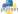# Python 3 - Magical Methods and Tricks with extremely useful methods

June 03, 2019This post will show some really awesome tricks in python.

## Get the power of a number

Example, get 9^3 (9 to the power 3)

``````a ** b

5 ** 3
# 125``````

## Get square root of a number

``num ** 0.5``

## Tricks with print() method

print() method always put a new line character in the end. You can change that behavior as well

``````# ends with space
print(n, end=" ")

# ends with comma and a space
print(n, end=", ")``````

## Count occurrence of words in a string

``````def count_words(line):
result = {}
words = line.split()
for word in words:
result[word] = result.get(word, 0) + 1

return result``````

Or, a more better way to calculate count of words:

``````from collections import Counter
def count_words(line):
return Counter(line.split())``````

## Magic with Sequences

``````# Example array
a = [1, 2, 3, 4, 5]

# Get first element
a

# Get last element
a[-1]

# Get element from 1st to 4th index
a[1:5]

# Get last 5 elements
a[-5:]

# First five elements
a[:5]

# Skip first 5 elements
a[5:]

# Get from index: 2 to 7, but skip by 2 index
a[2:8:2]

# Reverse copy of a list
a[::-1]

# Get even indexes starting from 1
a[1::2]``````

## Insert sub-array in between an array

``````a = [10, 20, 30, 40, 50]

# Insert [1, 2, 3, 4] after index=1
a[1:1] = 1, 2, 3, 4
print(a)
[10, 1, 2, 3, 4, 20, 30, 40, 50]``````

Also, you can replace some indexes too

``````a = [10, 20, 30, 40, 50]

# Insert [1, 2, 3, 4] after index=2, and replace indexes=2,3
a[2:4] = 1, 2, 3, 4
print(a)
[10, 20, 1, 2, 3, 4, 50]``````

Replace sequence of indexes to a single number

``````a[2:7] = 778

i.e. replace indexes=2, 3, 4, 5, 6 and insert number 778``````

## Insert in array at some position, and shift rest of array

Note: Above method replaces value at index specified. To just insert values, use below method

``````a = [10, 20, 30, 40, 50]
# Insert 90 at index=1
a.insert(1, 90)
print(a)
[10, 90, 20, 30, 40, 50]``````

## Find an Odd number

No, I’m not showing n%2==0 kind of method

``````a = [1, 2, 3, 4, 5]
[ v for v in a if v & 1 ]

print(a)
[1, 3, 5]``````

## Square of Odd numbers

``````a = [1, 2, 3, 4, 5]
[ v*v for v in a if v & 1 ]

print(a)
[1, 9, 25]``````

## Capitalize all names which starts with letter ‘r’ (for example)

``````users = ["virat kohli", "ajay jadeja", "rohit sharma", "ram sharma"]

[ n.capitalize() for n in users if n in 'rR']

['Rohit sharma', 'Ram sharma']``````

## Create a dictionary of number and its square

``````a = [10, 20, 30]
{ v: v*v for v in a}
{10: 100, 20:400, 30:900}``````

## Get a tuple of square

``````a = [10, 20, 30]

tuple(x*x for x in a)

(100, 400, 900)``````